CAUSAL MEDIATION ANALYSIS

MEDIATE IN ACTION!

Researchers may wish to find out whether physical exercise leads to an improvement in self-perceived well-being, and if so, to what extent. In addition, they want to learn more about the mechanisms through which such causal effects operate. Perhaps exercising causes an increase in certain chemicals or hormones in the human body, which in turn affects perceptions of well-being. To answer questions such as these, one use a causal mediation model to estimate the average treatment effect and decompose it into direct and indirect effects.

Fictional data from a randomized controlled trial is at hand, with individuals randomized into two groups—one group performs physical exercise, and the other group spends the same amount of time in a resting state. Subjective well-being is measured before and after treatment sessions. In addition, the level of the (fictional) hormone bonotonin is measured. Researchers wish to determine whether exercise leads to an increase in bonotonin levels, which in turn has a positive effect on subjective well-being.

One can use a binary outcome variable (wellbeing) that indicates an increase in well-being of at least 10% compared with a baseline measurement. The mediator variable (bonotonin) is also binary and measures whether there was an increase of at least 10% in the production of this chemical.

Using a logit model for both outcome and mediator, one can specify the following causal mediation model: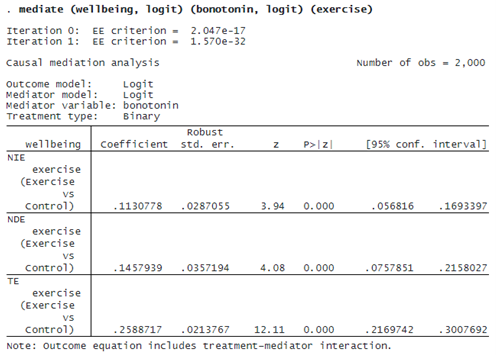The estimated total effect (TE) is 0.26. Because our outcome variable is binary, this effect is measured on the probability scale. We interpret this effect just like an average treatment effect: if every individual in the population would exercise, the probability of a higher well-being would increase by 0.26 points on the probability scale compared with if no one exercised.

The remaining results shown in the table are the estimates of the natural indirect effect (NIE) and natural direct effect (NDE). The NIE tells us to what extent exercise affects well-being through the production of bonotonin. The NDE captures the effect of exercise on well-being through mechanisms other than bonotonin. Here the NIE of 0.11 means that a 0.11 increase in the probability of higher well-being is due to the effect of exercise via the production of bonotonin. And based on the NDE, an increase of 0.15 is due to other mechanisms.

Above, we interpret the estimated effects on the probability scale as risk differences. If we wanted, we could also interpret them in terms of risk ratios or odds ratios. To get odds ratios, for instance, we use the postestimation command estat or: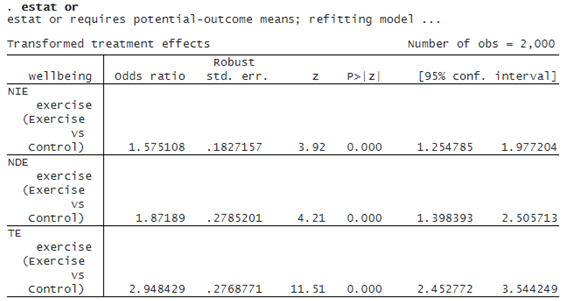The total effect corresponds to an odds ratio of 2.95, which in this case is the product of the direct- and indirect-effect odds ratios. To compute risk ratios, we could use estat rr, and had we fit a Poisson model for the outcome, we could have used estat irr to compute incidence-rate ratios.

We can also estimate controlled direct effects (CDE) using estat cde. For example, we could be interested in the direct effect under the counterfactual assumption that either every individual in the population experiences an increase in bonotonin levels or no one does. To do so, we specify estat cde with option mvalue(0 1) to estimate the average controlled direct effect of the treatment with the (binary) mediator bonotonin set to either 0 or 1: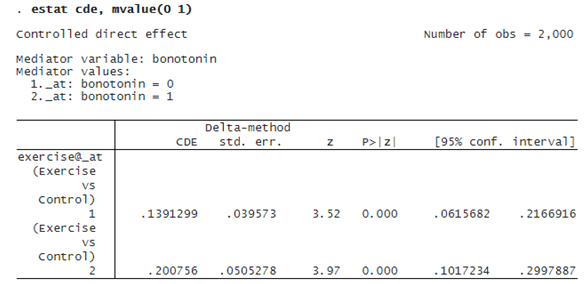The direct effect of exercise on an increase in well-being is 0.14 on the probability scale if no one experiences an increase in bonotonin levels, and we can see that the effect is 0.2 if everyone in the population were to experience an increase in bonotonin levels.

Again, if we wanted to express these effects in terms of risk ratios or odds ratios, we can use options rr or or, respectively. Here we use option or to estimate controlled direct effects on the odds-ratio scale: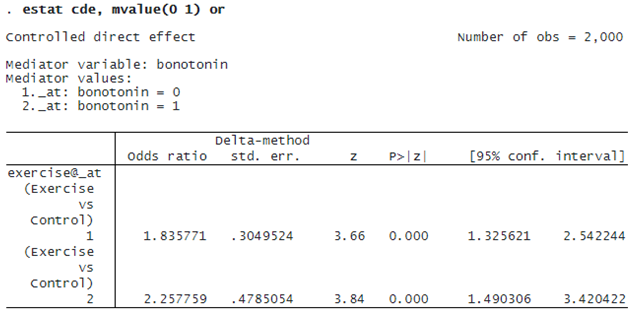Finally, we might be interested in assigning a number to the amount of mediation. We can use estat proportion to compute the proportion mediated: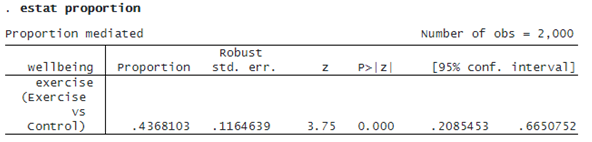The estimated proportion is based on the effect estimates on the probability scale. The indirect effect accounts for 44% of the total effect.